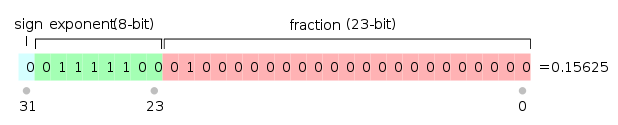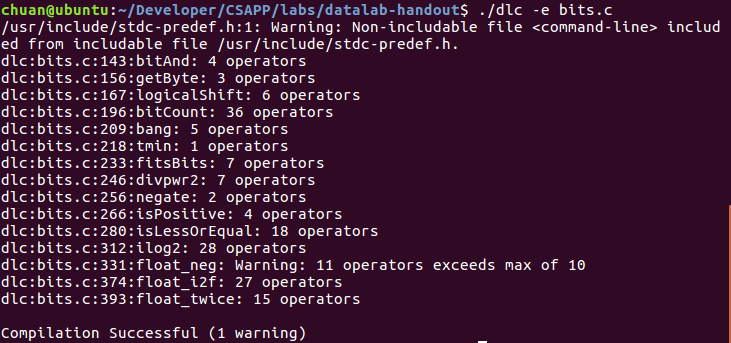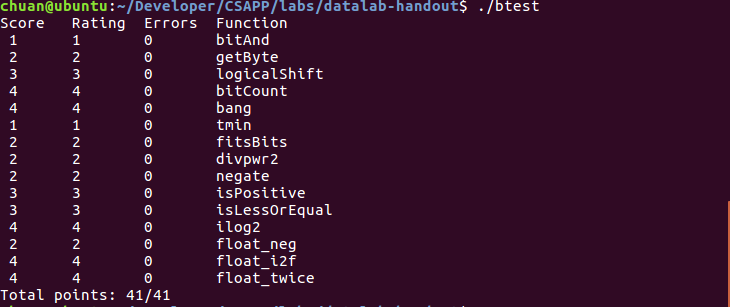0%

## 总体要求

1. 使用的整数必须在 0~255(0xFF)之间，而不允许使用大的常量，如：0xFFFFFFFF
2. 不可以使用全局变量
3. 在整数上可以使用的一元运算符只有：! 和 ~
4. 在整数上可以使用的二元运算符只有：& ^ | + << >>
5. 对个别题目的限制可能会略微放宽，如允许使用循环
6. 每个答案会限制允许的最大操作数
7. 在完成实验后，可以使用 dlc 工具检查代码的格式和操作数是否符合要求，使用 btest 检查结果是否通过测试。

## 题目及思路

### bitAnd

• 要求：计算 x & y 的值
• 允许操作：~ |
• 操作数限制：8次

$$(x & y) = (x) | (y) ==> (x & y) = ~((x) | (~y))$$

### getByte

• 要求：得到 x 中第 n 个字节
• n 的范围：[0, 3]
• 允许操作：! ~ & ^ | + << >>
• 操作数限制：6

Endianness only matters for layout of data in memory. As soon as data is loaded by the processor to be operated on, endianness is completely irrelevent. Shifts, bitwise operations, and so on perform as you would expect (data logically laid out as low-order bit to high) regardless of endianness

### logicalShift

• 要求：将 x 逻辑右移 n 位
• 假定 0 <= n <= 31
• 允许操作：! ~ & ^ | + << >>
• 操作数限制：20

### bitCount

• 要求：得到在某个字中为 1 的 bit 个数
• 允许操作：! ~ & ^ | + << >>
• 操作数限制：40

### bang

• 要求：计算 !x
• 允许操作：~ & ^ | + << >>
• 操作数限制：12

### tmin

• 要求：返回使用2进制补码表示的最小的整数
• 允许操作：! ~ & ^ | + << >>
• 操作数限制：4

### fitsBits

• 要求：如果x可以被 n 位的2进制补码表示则返回1，否则返回0
• 假定1 <= n <= 32
• 允许操作：! ~ & ^ | + << >>
• 操作数限制：15

n 位补码能够表示的数的范围是$[-2^{n-1}, 2^{n-1}-1]$，所以如果在这个范围内则返回1，否则返回0。如果x大于$2^{n-1}-1$，则会发生正溢出，得到负数；如果x小于$-2^{n-1}$则会发生负溢出。所以题目的求解方法就是将x的符号位从第 n 位拓展到第32位得到extened_x，之后通过判断x == exntened_x来判断是否发生了溢出。

### divpwr2

• 要求：计算$x/2^{n}$，并向零舍入
• 假定0 <= n <= 30
• 允许操作：! ~ & ^ | + << >>
• 操作数限制：15

### negate

• 要求：计算 -x
• 允许操作：! ~ & ^ | + << >>
• 操作数限制：5

### isPositive

• 要求：如果x > 0返回1，否则返回 0
• 允许操作：! ~ & ^ | + << >>
• 操作数限制：8

### isLessOrEqual

• 要求：如果x <= y返回 1，否则返回 0
• 允许操作：! ~ & ^ | + << >>
• 操作数限制：24

1. x < 0 并且 y > 0
2. x 和 y 的符号位相同，并且 x - y < 0
3. x = y

### ilog2

• 要求：计算$log_2^{x}$，向下舍入
• 允许操作：! ~ & ^ | + << >>
• 操作数限制：90

### float_neg

• 要求：f 为浮点数的位级表示，返回-f的位级表示，如果 f 为 NaN，返回 f
• 允许操作：允许所有有/无符号数的操作，并允许使用 if 和 while
• 操作数限制：10### float_i2f

• 要求：返回整数 x 的浮点数的位级表示
• 允许操作：允许所有有/无符号数的操作，并允许使用 if 和 while
• 操作数限制：30

(sign << 31) | (exponent) << 23 | fraction

1. 当 x = 0 时，返回 0
2. 当 x = 0x80000000 时，令 exponent = 158，因为 158 - 127 = 31，其中 Bias = $2^{n-1} - 1$
3. 如果 x < 0, 则 sign = 1；并令 x = -x，这样之后在做移位等操作就不用担心符号位了
4. 计算整数 x 的位数，作为计算 exponent 的依据
5. 得到 exponent 后，将 x 右移计算小数值 fraction，并且根据移除的值是否大于等于1.5来判断是否要进位，从而实现向零舍入
6. 如果小数位过多，则丢弃最高位并增加 exponent
7. 组合符号位、指数位和小数位

### float_twice

• 要求：返回 2 * f 的位级表示，f 为浮点数 f 的位级表示
• 如果 f 为 NaN 则返回 f
• 允许操作：允许所有有/无符号数的操作，并允许使用 if 和 while
• 操作数限制：30

1. 若 f 为+0-0，返回 f
2. 若 f 为无穷大或 NaN，返回 f
3. 若 f 为非规格化浮点数，则保留符号位，并将 f 左移1位
4. 若 f 为规格化浮点数，则将 f 的指数位增加1

## 最终结果